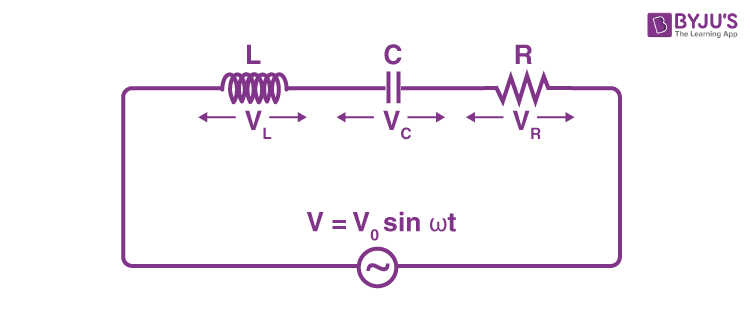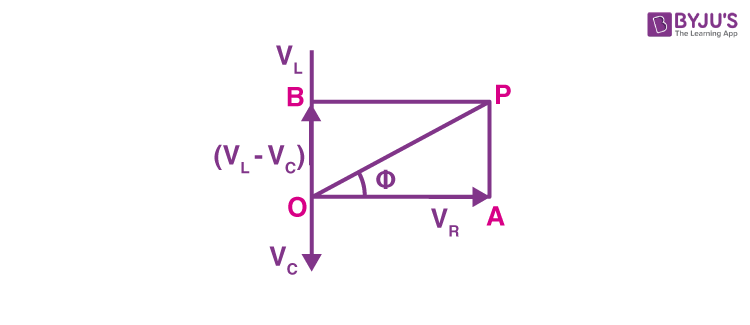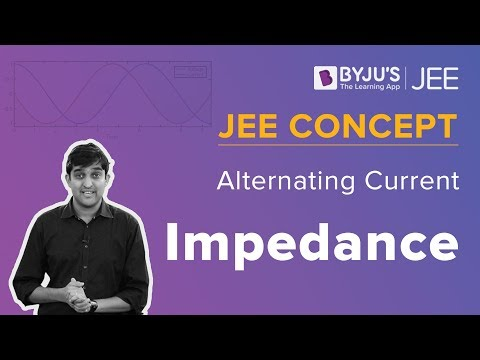Checkout JEE MAINS 2022 Question Paper Analysis : Checkout JEE MAINS 2022 Question Paper Analysis :

# Impedance Of A Series LCR Circuit

## What Is Impedance?

Impedance is defined as a combination of resistance and reactance. It is basically an obstruction to the flow of electrons in an electric circuit. Z is the symbol used for describing the impedance. The unit of impedance is the ohm.

The other way of defining impedance in terms of the phasor is that it is the ratio of the phasor voltage across the element to the phasor current through the element.

## What Is LCR Circuit?

The LCR circuit is defined as the circuit that consists of a resistor R, an inductor L, and a capacitor C. These three electrical components are either connected in series or in parallel. LCR circuit is also known as RLC circuit and this circuit gets its name from the symbols of the main electronic components that are used. The other names of the LCR circuit are resonant circuit and tuned circuit.

## Derivation Of The Expression For The Impedance Of A Series LCR Circuit

In the below circuit diagram, let R, L and C be the resistance, inductance, and capacitance that is connected in series with an alternating current source.The voltage applied across the LCR series circuit is given as:

v = vo sinωt

Where,

• v is the instantaneous vale
• vo is the peak value
• ω = 2πf
• f is the frequency of alternating current

Let i be the instantaneous current at the time t such that the instantaneous voltage across R, L, and C are iR, iXL, and iXC, respectively.

The vector sum of the voltage amplitudes across R, L, C and the amplitude vo of the applied voltage are equal.The voltage amplitudes across R, L, and C are given as vR, vL, and vC, respectively. The current amplitude is given as Io.

Then, vR = IoR is in the phase with io.

vL = ioXL = Io(⍵L)

The above equation leads io by 90o

vC = ioXC = io(1/⍵C)

The above equation lags behind io by 90o

We can notice that the current in a pure resistor is in phase with the voltage while the current in a pure inductor and the voltage lag by rad. The current in a pure capacitor and the voltage are led by rad.

When vL > vC, the phase angle (Φ) between the voltage and the current is positive.

OAP is the right-angled triangle from which we get,

OP2 = OA2 + AP2 = OA2 + OB2 (Q AP = OB)

v2 = v2R + (VL – VC)2 = (iR)2 + (iXL – iXC)2

= i2(R2 + (XL – XC)2)

Therefore,

$$\begin{array}{l}i=\frac{v}{\sqrt{R^{2}+(X_{L}-X_{C})^{2}}}=\frac{v}{Z}\end{array}$$
$$\begin{array}{l}Z=\sqrt{R^{2}+(X_{L}-X_{C})^{2}}\end{array}$$

Here, Z is the impedance of the circuit

The phase angle between v and i is given as:

$$\begin{array}{l}tan \phi =\frac{v_{L}-v_{c}}{v_{R}}=\frac{X_{L}-X_{C}}{R_{L}}\end{array}$$

This gives us:

$$\begin{array}{l}\phi =tan^{-1}\frac{X_{L}-X_{C}}{R}\end{array}$$

The below video is an explanation of alternating current and impedance. Please refer to the video for a better understanding of the concepts:## Frequently Asked Questions – FAQs

### What is meant by impedance?

Impedance is defined as a combination of resistance and reactance. It is basically an obstruction to the flow of electrons in an electric circuit.

### What is the unit of impedance?

The unit of impedance is the ohm.

### What is a series circuit?

A series circuit consists of a path along which the entire current travels through every individual component.

### What are the main two types of the electrical circuit?

Series circuits and parallel circuits are the main two types of the electrical circuit.

### What is an LCR circuit?

The LCR circuit is defined as the circuit that consists of a resistor R, an inductor L, and a capacitor C. These three electrical components are either connected in series or in parallel. LCR circuit is also called an RLC circuit.